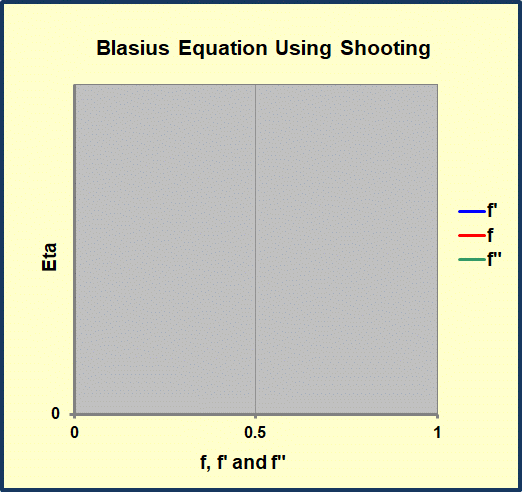# Solution of Blasius Equation Using Shooting

UPDATED: 08/25/2022

This workbook performs a numerical solution of the Blasius equation for flow in a laminar, self-similar, flat plate boundary layer. The Runge-Kutta integration scheme and shooting algorithm used to solve this third-order, non-linear, ordinary differential equation were taken from An Introduction to Computational Fluid Mechanics by C.Y. Chow and embellished with Excel graphics.  Once the value of f'(∞) is close to the desired value (1.0), a half-interval method is used to correct f”(0) until the converged value is found.   Tabulated results from a classical source (Howarth’s results as reported in Schlicting) are included for comparison with the current solution.Shooting solution of Blasius Equation for flow in a laminar boundary layer. Shows f, f’ (velocity), and f” (shear) for a sequence of shots. Eventually the outer boundary condition f’ = 1.0 is matched.

The “.332” appearing in the correlation for laminar, forced convection on a flat plate follows directly from the value of f”(0) found eventually in the above calculation.